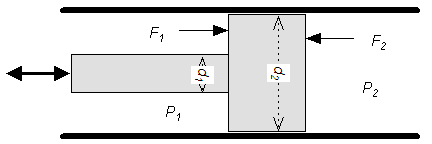## Hydraulic Force calculator

### Pressure on Rod Side

piston diameter (in)
rod diameter (in)
cyl. pressure (psi)

piston diameter (mm)
rod diameter (mm)
cyl. pressure (bar)

### Pressure opposite Rod Side

piston diameter (in)
cyl. pressure (psi)

piston diameter (mm)
cyl. pressure (bar)### The force produced by a double acting hydraulic piston on the rod side formula

F1 = π / 4 (d22 - d12) P1

where

d2 = piston diameter (inches)

d1 = rod diameter (inches)

F1 = rod pull force (lb)

P1 = pressure in the cylinder (rod side) (lff/in2)

### The force produced opposite the rod formula

F2 = π / 4 d22 P2

where

P2 = pressure in the cylinder (opposite rod) (lff/in2)

F2 = rod push force (lb)

psi (lb/in2) = 144 psf (lbf/ft2) = 6,894.8 Pa (N/m2) = 6.895x10-3 N/mm2 = 6.895x10-2 bar

1 lbf (Pound force) = 4.44822 N = 0.4536 kp

1 in (inch) = 25.4 mm

Thinkcalculator.com provides you helpful and handy calculator resources.